Question

Determine the pH of each of the following solutions.

Determine the pH of each of the following solutions.

Part A

0.21 MKCHO2

Part B

0.17 MCH3NH3I

Part C

0.23 MKI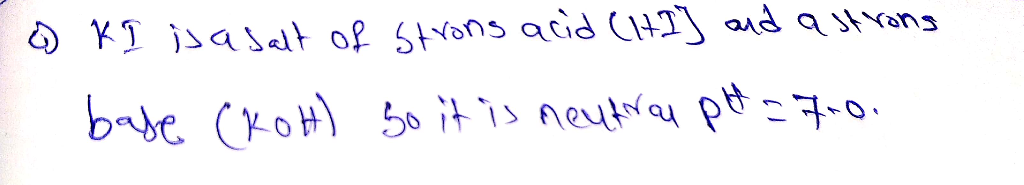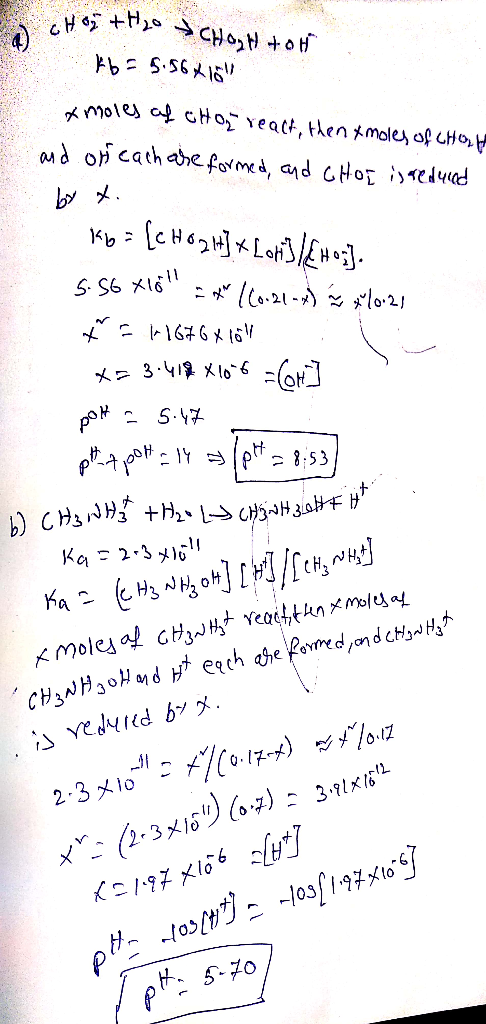Earn Coins

Coins can be redeemed for fabulous gifts.

Similar Homework Help Questions
• Determine the pH of each of the following solutions. Determine the pH of each of the...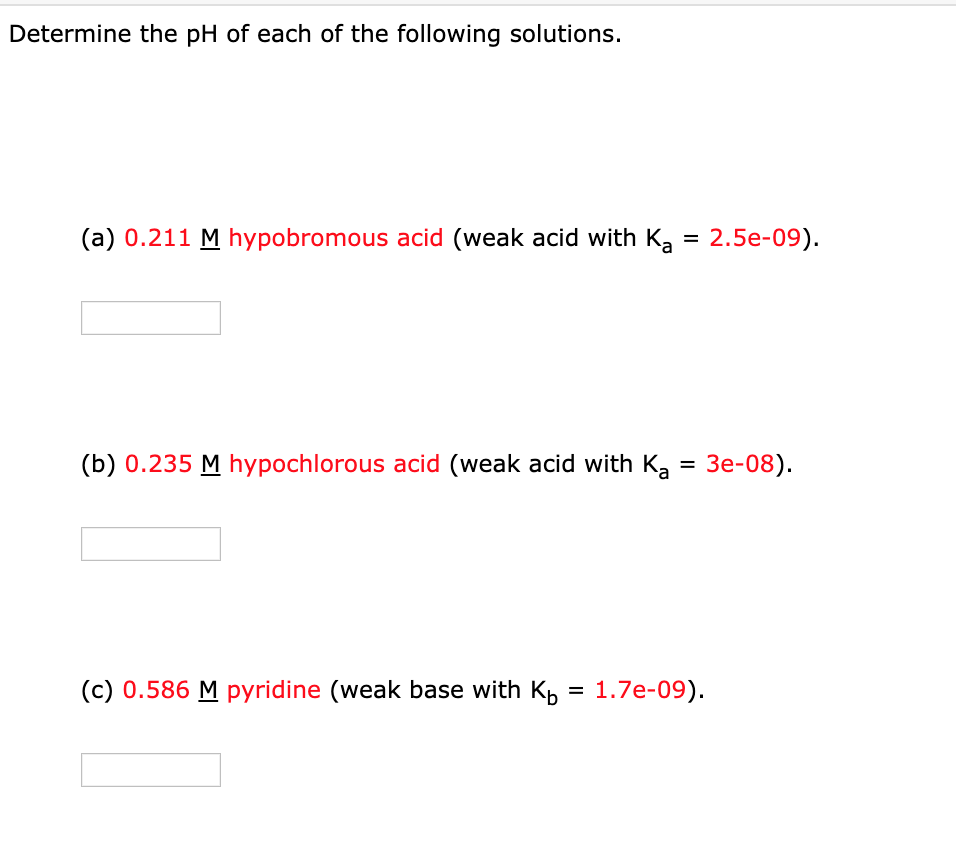Determine the pH of each of the following solutions. Determine the pH of each of the following solutions. (a) 0.211 M hypobromous acid (weak acid with Ka = 2.5e-09) Ка 3 Зе-08). (b) 0.235 M hypochlorous acid (weak acid with Ka (c) 0.586 M pyridine (weak base with K 1.7e-09)

• Please show steps. Thanks. Determine the pH of each of the following solutions. 0.21 M CH3NH3I...Please show steps. Thanks. Determine the pH of each of the following solutions. 0.21 M CH3NH3I pH = 11.97

• Determine the pH of each of the following solutions. 0.13 M NH4Cl 0.11 M NaC2H3O2 0.17...

Determine the pH of each of the following solutions. 0.13 M NH4Cl 0.11 M NaC2H3O2 0.17 M NaCl Express your answer to two decimal places.

• 1. Calculate the pH of each of the following solutions and determine whether the solutions are...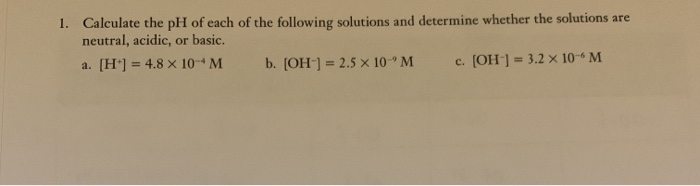1. Calculate the pH of each of the following solutions and determine whether the solutions are neutral, acidic, or basic. a. [H] = 4.8 x 10-M b. [OH-] = 2.5 X 10M c. [OH-] = 3.2 X 10M

• Determine the pH of each of the following solutions. Part A 0.11 molL−1 NH4Cl Express your...

Determine the pH of each of the following solutions. Part A 0.11 molL−1 NH4Cl Express your answer to two decimal places. Part B 0.20 molL−1 CH3COONa Part C 0.19 molL−1 NaCl

• UN CUNCILIULUNU 64. Calculate the pH and the pOH of each of the following solutions at...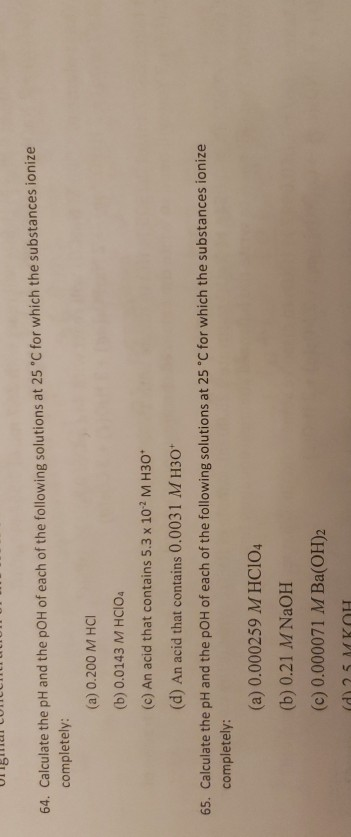UN CUNCILIULUNU 64. Calculate the pH and the pOH of each of the following solutions at 25 C for which the substances ionize completely: (a) 0.200 M HCI (b) 0.0143 M HCIO (c) An acid that contains 5.3 x 102 M H30* (d) An acid that contains 0.0031 M H30 65. Calculate the pH and the pOH of each of the following solutions at 25°C for which the substances ionize completely: (a) 0.000259 M HCIO4 (b) 0.21 M NaOH (c)...

• Determine the pH of each of the following solutions. Part A 0.20 molL−1 NH4Cl Express your...

Determine the pH of each of the following solutions. Part A 0.20 molL−1 NH4Cl Express your answer to two decimal places. Part B 0.11 molL−1 CH3COONa Express your answer to two decimal places. Part C 0.16 molL−1 NaCl

• Determine the pH of each of the following solutions. part b) 0.11 molL−1 CH3COONa part c) 0.18 molL−1 NaCl

Determine the pH of each of the following solutions. part b) 0.11 molL−1 CH3COONa part c) 0.18 molL−1 NaCl

• Determine the pH of each of the following solutions. A, B, C, D, E Part A...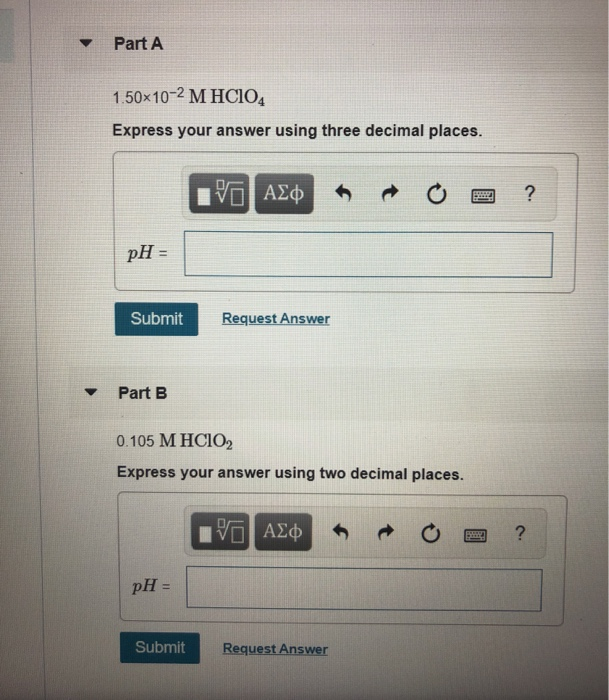Determine the pH of each of the following solutions. A, B, C, D, E Part A 1.50x10-2 M HC104 Express your answer using three decimal places. IV ALQ R 0 = ? pH = Submit Request Answer Part B 0.105 M HClO2 Express your answer using two decimal places. VALD ROO? pH = Submit Request Answer Part C 4.5x10-2 M Sr(OH)2 Express your answer using two decimal places. IVO ALO O O ? pH- Submit Request Answer Part D 8...

• Determine the pH of each of the following solutions. Part A 0.11 molL−1 NH4Cl Express your...

Determine the pH of each of the following solutions. Part A 0.11 molL−1 NH4Cl Express your answer to two decimal places. Part B 0.15 molL−1 CH3COONa Express your answer to two decimal places. Part C 0.13 molL−1 NaCl Express your answer to two decimal places.